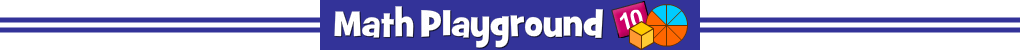Sailboat Subtraction
NUMBER OF PLAYERS: 8
Do you know subtraction facts through 12? Can you subtract numbers in your head?
Practice this important skill and move your sailboat to victory.
Sailboat Subtraction - Learning Connections
Essential Skills
Mental Math - fast recall of subtraction facts
Problem Solving - match mathematical expressions with the correct value

Common Core Connection for Grades 1 and 2
Understand subtraction as an unknown-addend problem.
Apply properties of operations as strategies to subtract.
Subtract within 20.
More Math Games to Play